# pygmt.Figure

class pygmt.Figure[source]

A GMT figure to handle all plotting.

Use the plotting methods of this class to add elements to the figure. You can preview the figure using pygmt.Figure.show and save the figure to a file using pygmt.Figure.savefig.

Unlike traditional GMT figures, no figure file is generated until you call pygmt.Figure.savefig or pygmt.Figure.psconvert.

Examples

>>> import pygmt
>>> fig = pygmt.Figure()
>>> fig.basemap(region=[0, 360, -90, 90], projection="W15c", frame=True)
>>> fig.savefig("my-figure.png")
>>> # Make sure the figure file is generated and clean it up
>>> import os
>>> os.path.exists("my-figure.png")
True
>>> os.remove("my-figure.png")


The plot region can be specified through ISO country codes (for example, "JP" for Japan):

>>> import pygmt
>>> fig = pygmt.Figure()
>>> fig.basemap(region="JP", projection="M7c", frame=True)
>>> # The fig.region attribute shows the WESN bounding box for the figure
>>> print(", ".join(f"{i:.2f}" for i in fig.region))
122.94, 145.82, 20.53, 45.52


Methods Summary

 Figure.basemap(*[, region, projection, ...]) Plot base maps and frames for the figure. Figure.coast(*[, region, projection, ...]) Plot continents, shorelines, rivers, and borders on maps. Figure.colorbar(*[, region, projection, ...]) Plot a gray or color scale-bar on maps. Figure.contour([data, x, y, z, annotation, ...]) Contour table data by direct triangulation. Figure.grdcontour(grid, *[, annotation, ...]) Convert grids or images to contours and plot them on maps. Figure.grdimage(grid, *[, img_out, frame, ...]) Project and plot grids or images. Figure.grdview(grid, *[, region, ...]) Create 3-D perspective image or surface mesh from a grid. Figure.histogram(data, *[, horizontal, ...]) Plots a histogram, and can read data from a file or list, array, or dataframe. Figure.image(imagefile, *[, region, ...]) Place images or EPS files on maps. Figure.inset(*[, position, box, projection, ...]) Create an inset figure to be placed within a larger figure. Figure.legend([spec, position, box, region, ...]) Plot legends on maps. Figure.logo(*[, region, projection, ...]) Plot the GMT logo. Figure.meca(spec, scale[, convention, ...]) Plot focal mechanisms. Figure.plot([data, x, y, size, direction, ...]) Plot lines, polygons, and symbols in 2-D. Figure.plot3d([data, x, y, z, size, ...]) Plot lines, polygons, and symbols in 3-D. Figure.psconvert([icc_gray, crop, ...]) Convert [E]PS file(s) to other formats. Figure.rose([data, length, azimuth, sector, ...]) Plot windrose diagrams or polar histograms. Figure.savefig(fname[, transparent, crop, ...]) Save the figure to a file. Figure.set_panel([panel, fixedlabel, ...]) Set the current subplot panel to plot on. Figure.shift_origin([xshift, yshift]) Shift plot origin in x and/or y directions. Figure.show([dpi, width, method, waiting]) Display a preview of the figure. Figure.solar([terminator, ...]) Plot day-light terminators or twilights. Figure.subplot([nrows, ncols, figsize, ...]) Create multi-panel subplot figures. Figure.ternary(data[, alabel, blabel, ...]) Reads (a,b,c[,z]) records from data and plots symbols at those locations on a ternary diagram. Figure.text([textfiles, x, y, position, ...]) Plot or typeset text strings of variable size, font type, and orientation. Figure.velo([data, vector, frame, cmap, ...]) Plot velocity vectors, crosses, anisotropy bars, and wedges. Figure.wiggle([data, x, y, z, frame, ...]) Plot z=f(x,y) anomalies along tracks.

## Examples using pygmt.Figure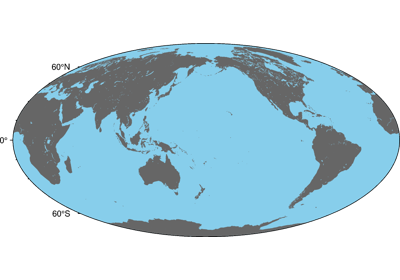Color land and water

Color land and water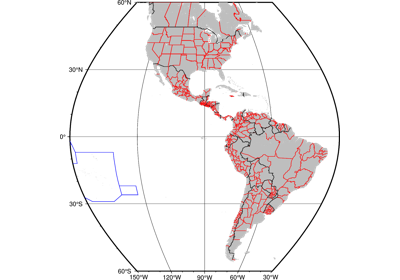Political Boundaries

Political BoundariesShorelines

Shorelines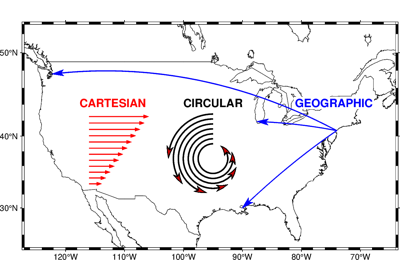Cartesian, circular, and geographic vectors

Cartesian, circular, and geographic vectors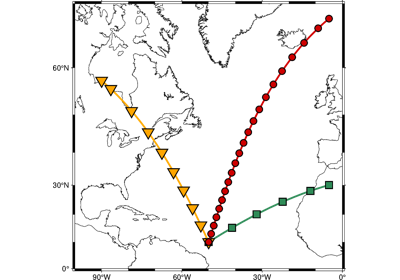Generate points along great circles

Generate points along great circles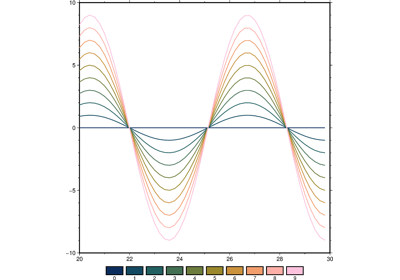Line colors with a custom CPT

Line colors with a custom CPT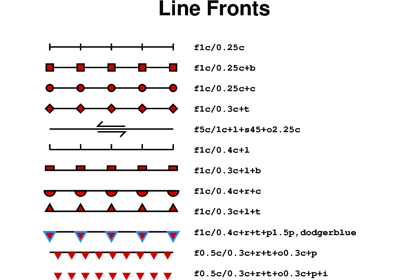Line fronts

Line fronts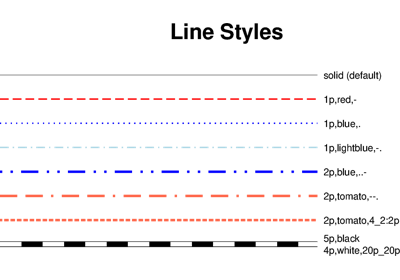Line styles

Line styles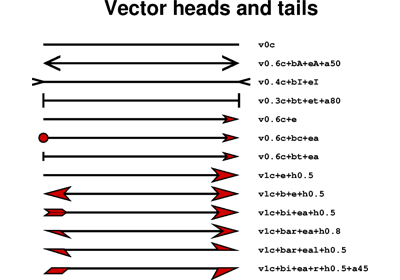Vector heads and tails

Vector heads and tails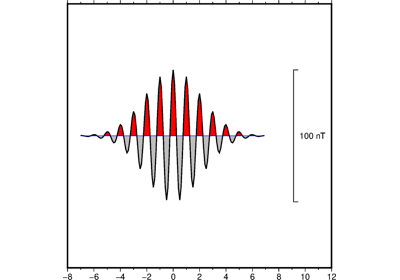Wiggle along tracks

Wiggle along tracks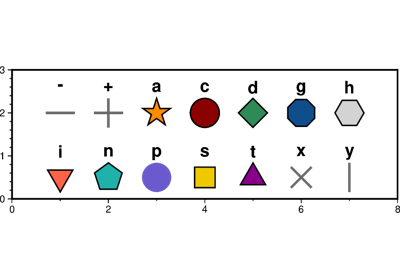Basic geometric symbols

Basic geometric symbols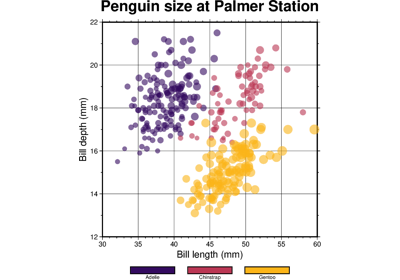Color points by categories

Color points by categoriesCustom symbols

Custom symbols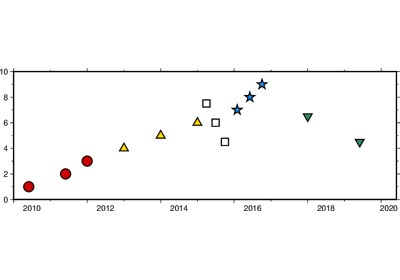Datetime inputs

Datetime inputs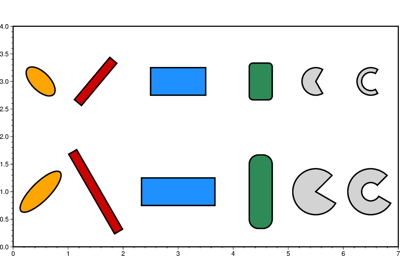Multi-parameter symbols

Multi-parameter symbolsPoints

Points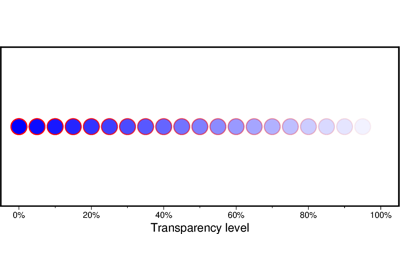Points with varying transparency

Points with varying transparency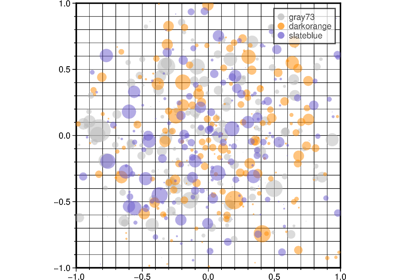Scatter plots with a legend

Scatter plots with a legend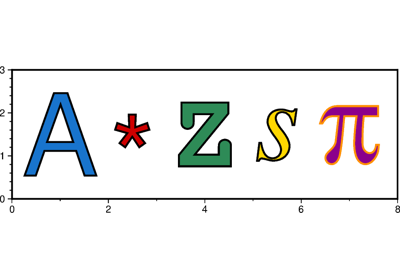Text symbols

Text symbols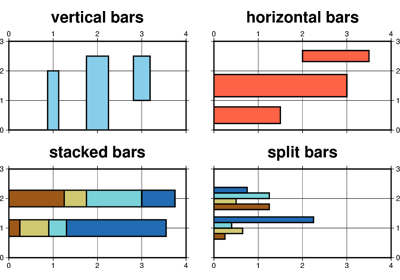Vertical or horizontal bars

Vertical or horizontal bars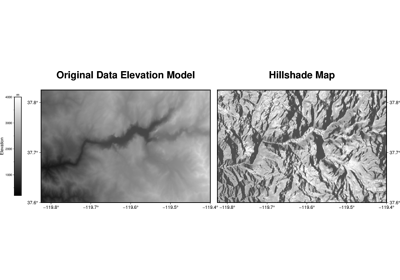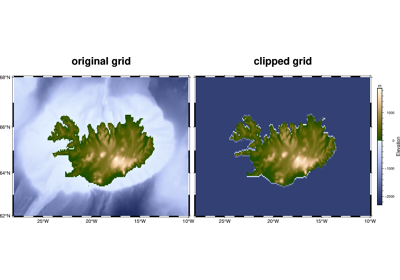Clipping grid values

Clipping grid valuesContours

Contours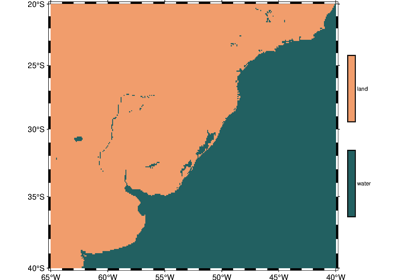Create ‘wet-dry’ mask grid

Create 'wet-dry' mask grid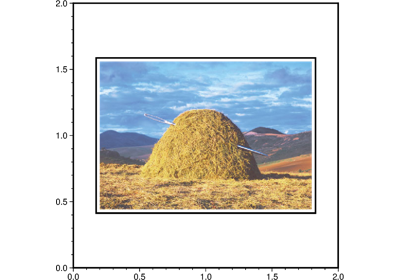Images on figures

Images on figures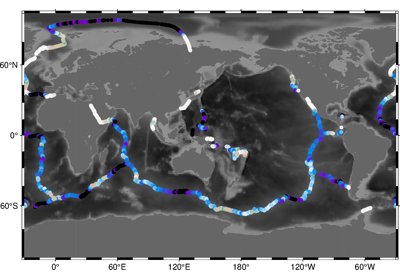Sampling along tracks

Sampling along tracks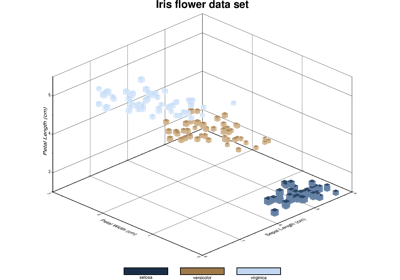3D Scatter plots

3D Scatter plots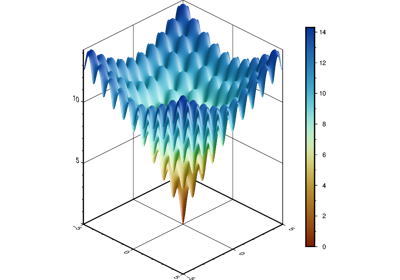Plotting a surface

Plotting a surfaceFocal mechanisms

Focal mechanisms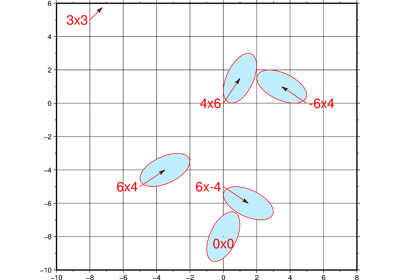Velocity arrows and confidence ellipses

Velocity arrows and confidence ellipses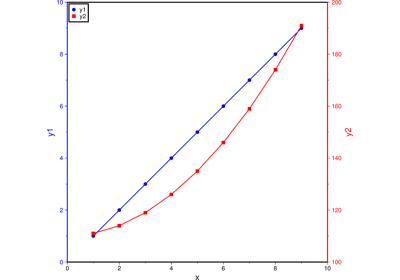Double Y axes graph

Double Y axes graph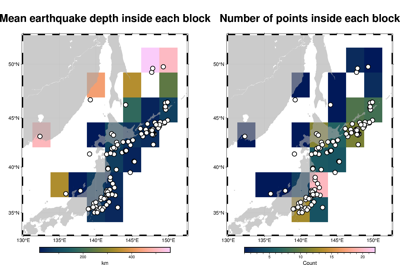Blockmean

Blockmean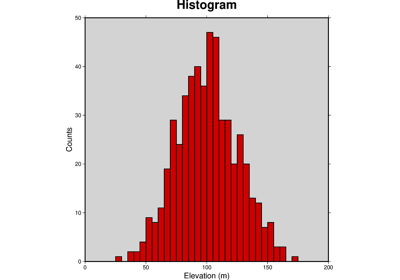Histogram

Histogram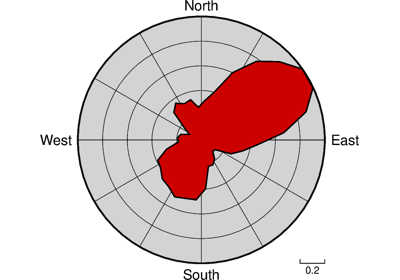Rose diagram

Rose diagramColorbar

Colorbar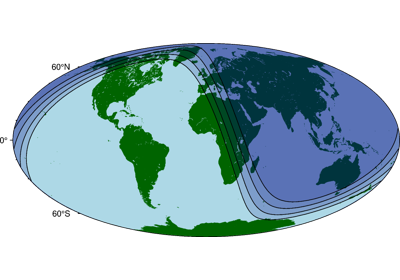Day-night terminator line and twilights

Day-night terminator line and twilights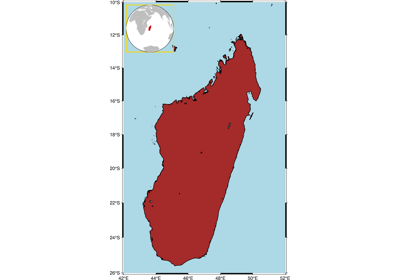Inset

Inset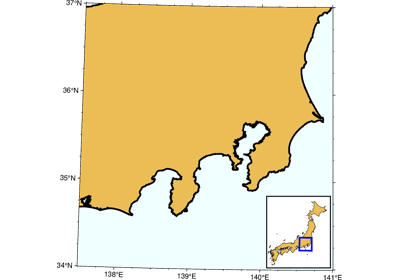Inset map showing a rectangular region

Inset map showing a rectangular region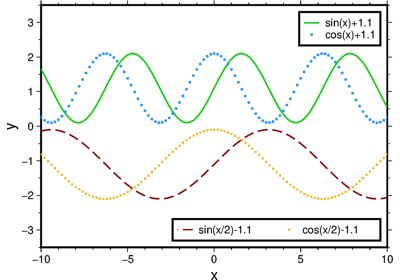Legend

LegendLogo

Logo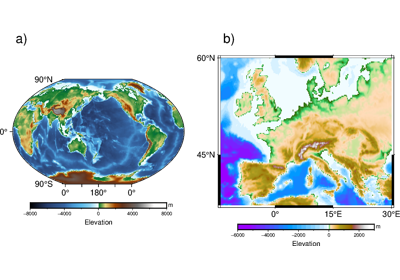Multiple colormaps

Multiple colormapsCoastlines and borders

Coastlines and borders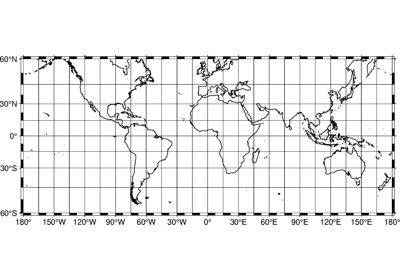Frames, ticks, titles, and labels

Frames, ticks, titles, and labels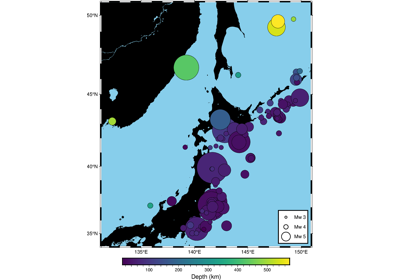Plotting data points

Plotting data pointsPlotting lines

Plotting lines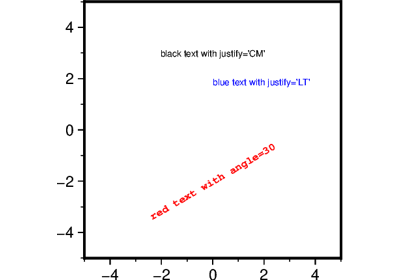Plotting text

Plotting text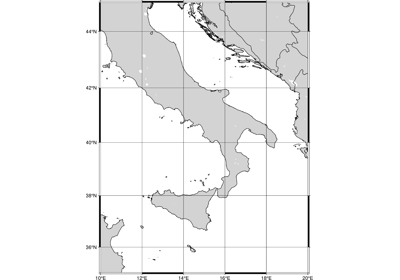Setting the region

Setting the region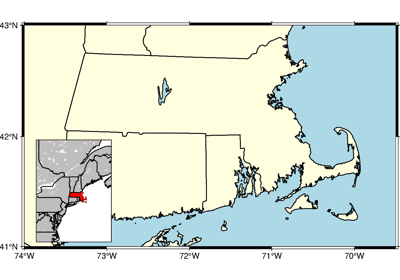Adding an inset to the figure

Adding an inset to the figure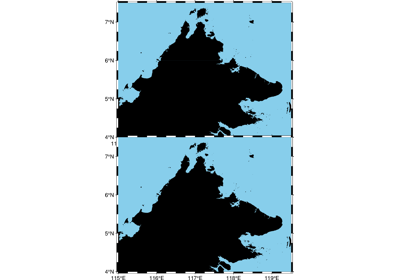Configuring PyGMT defaults

Configuring PyGMT defaults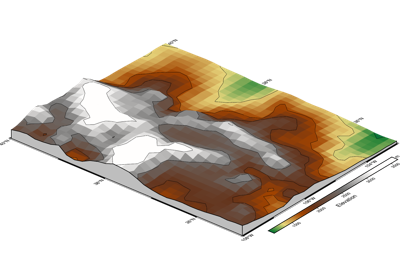Creating a 3D perspective image

Creating a 3D perspective image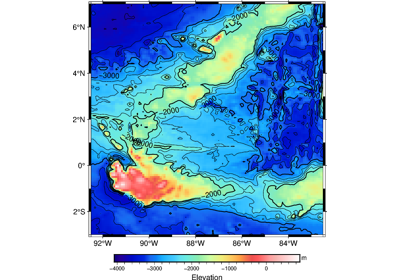Creating a map with contour lines

Creating a map with contour linesMaking subplots

Making subplots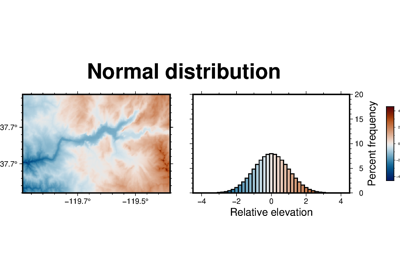Performing grid histogram equalization

Performing grid histogram equalization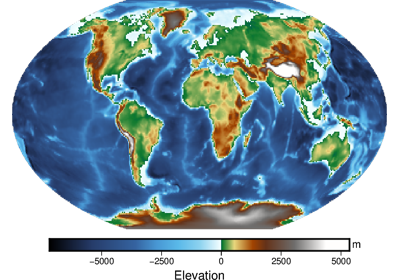Plotting Earth relief

Plotting Earth relief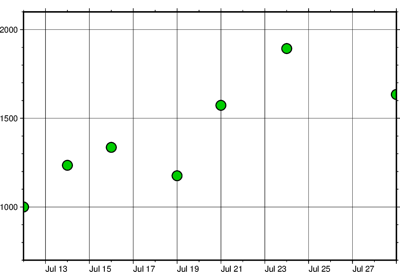Plotting datetime charts

Plotting datetime charts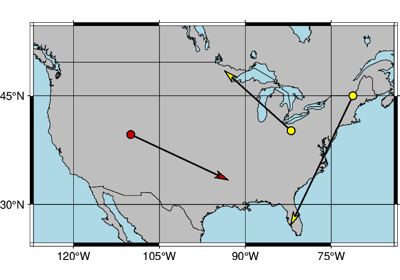Plotting vectors

Plotting vectors1. Making your first figure

1. Making your first figure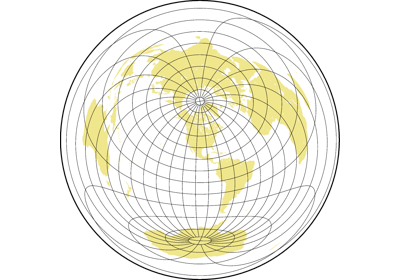Azimuthal Equidistant

Azimuthal Equidistant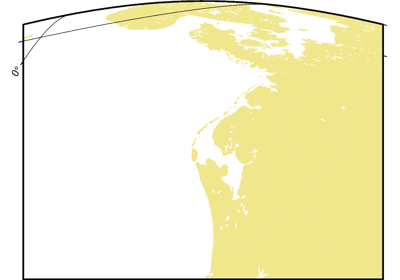General Perspective

General Perspective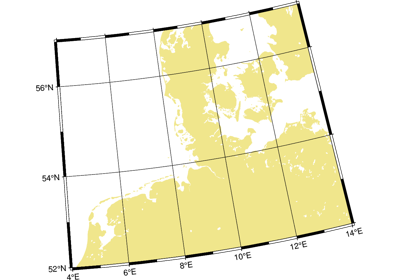General Stereographic

General Stereographic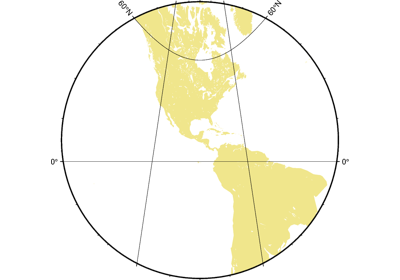Gnomonic

Gnomonic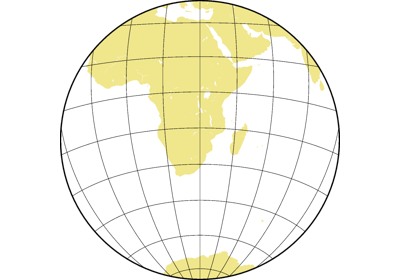Lambert Azimuthal Equal Area

Lambert Azimuthal Equal Area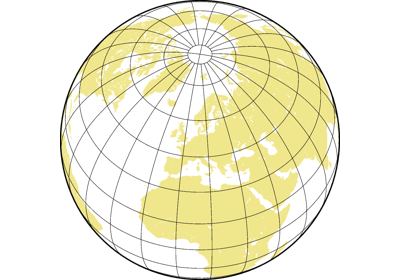Orthographic

Orthographic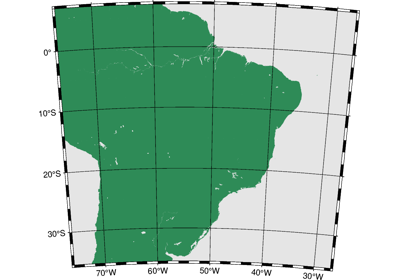Albers Conic Equal Area

Albers Conic Equal Area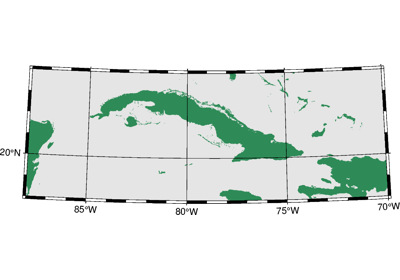Equidistant conic

Equidistant conic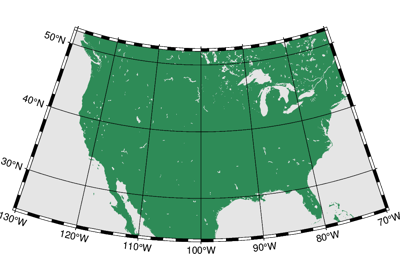Lambert Conic Conformal Projection

Lambert Conic Conformal Projection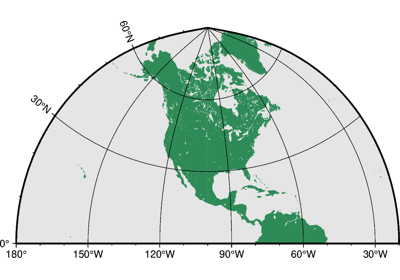Polyconic Projection

Polyconic Projection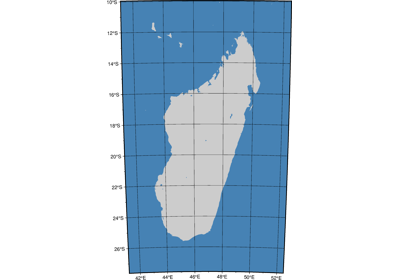Cassini Cylindrical

Cassini Cylindrical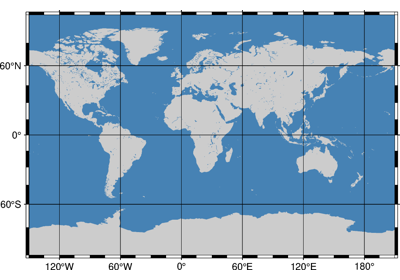Cylindrical Stereographic

Cylindrical Stereographic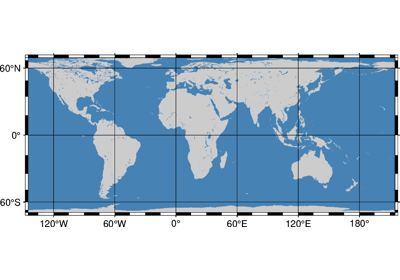Cylindrical equal-area

Cylindrical equal-area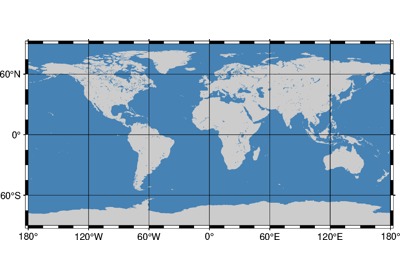Cylindrical equidistant

Cylindrical equidistant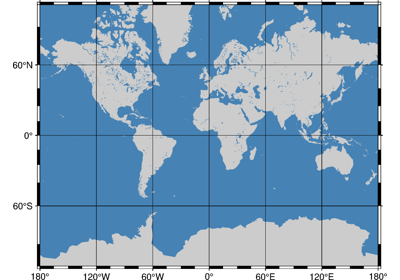Mercator

Mercator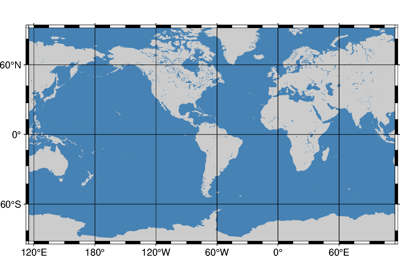Miller cylindrical

Miller cylindricalOblique Mercator, 1: origin and azimuth

Oblique Mercator, 1: origin and azimuthOblique Mercator, 2: two points

Oblique Mercator, 2: two pointsOblique Mercator, 3: origin and pole

Oblique Mercator, 3: origin and pole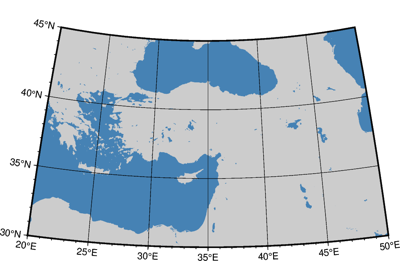Transverse Mercator

Transverse Mercator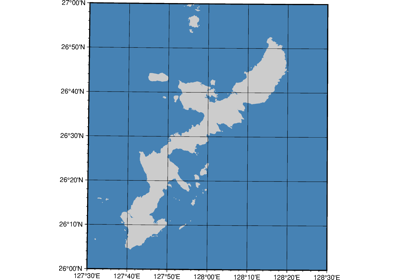Universal Transverse Mercator

Universal Transverse Mercator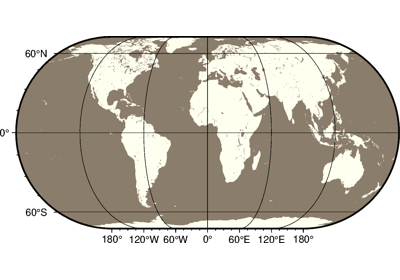Eckert IV

Eckert IV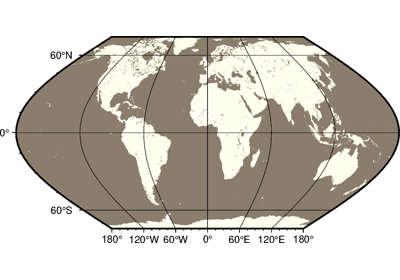Eckert VI

Eckert VI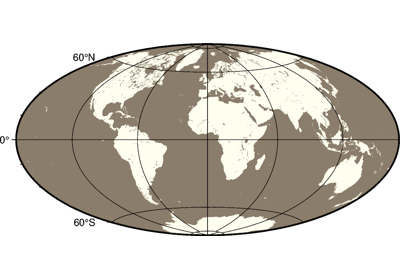Hammer

Hammer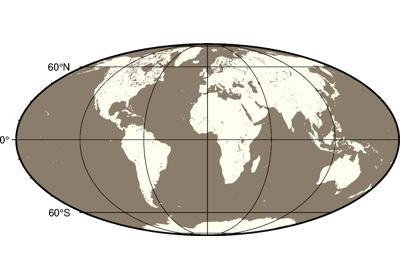Mollweide

Mollweide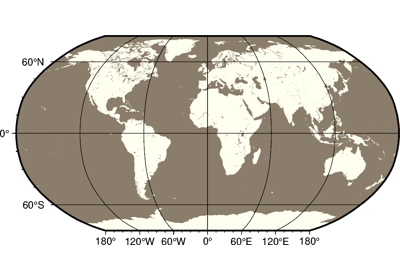Robinson

Robinson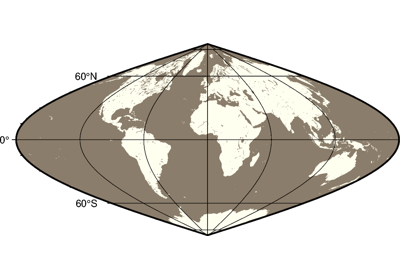Sinusoidal

Sinusoidal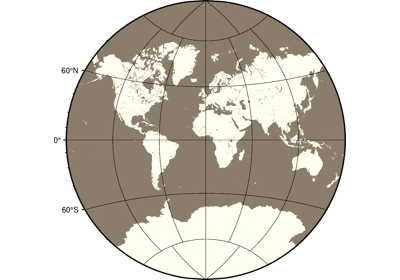Van der Grinten

Van der Grinten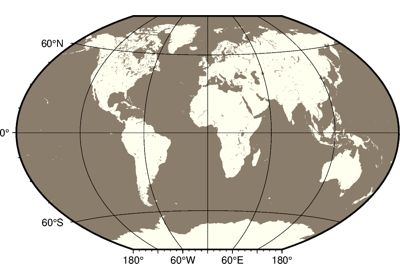Winkel Tripel

Winkel Tripel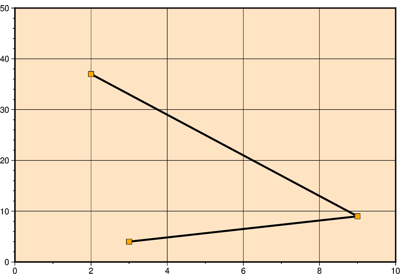Cartesian linear

Cartesian linear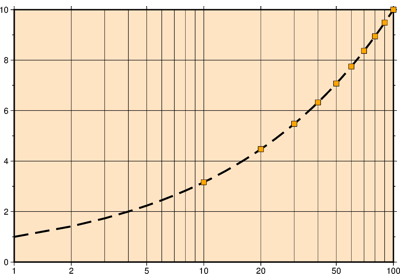Cartesian logarithmic

Cartesian logarithmic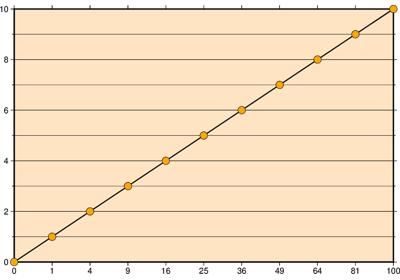Cartesian power

Cartesian power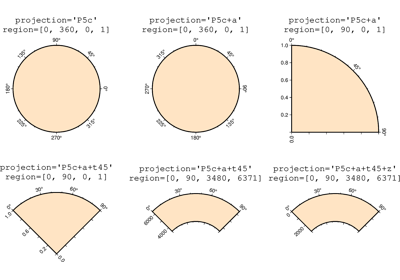Polar

Polar